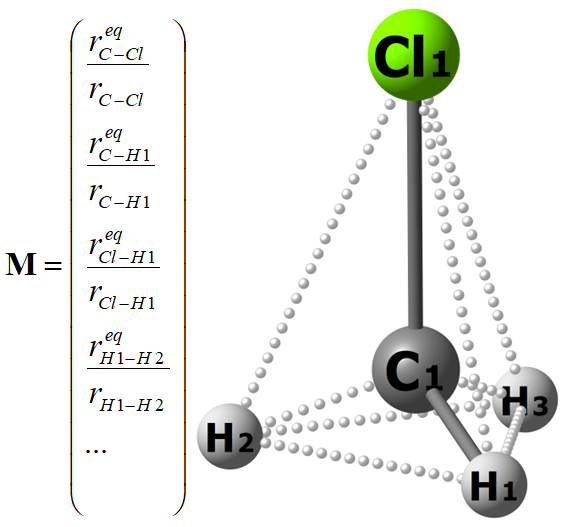# Self-Correcting Machine Learning and Structure-Based SamplingStructure-based sampling and self-correcting machine learning is used for precise representation of molecular potential energy surfaces and calculating vibrational levels with spectroscopic accuracy (errors less than 1 cm−1 relative to the reference ab initio spectrum) decreasing the number of required quantum mechanical calculations by up to 90%.

Machine learning (ML) is a great way to decrease the number of required costly quantum mechanical (QM) calculations. Nevertheless, ML models are not strictly based on any physically sound model. Thus, they tend to fail miserably when they encounter a situation they have not been trained for. This often leads to huge unphysical outliers, which are much larger than outliers obtained even with low-level semiempirical QM methods. Earlier we have proposed several ways to mitigate above problem by combining ML and low-level QM into the hybrid QM/ML methods, where low-level QM method is a baseline and also serves a role of a fail-safe. Our automatic parameterization technique and Δ-ML not only significantly decrease errors of the low-level QM methods, but also largely avoid huge unphysical outliers.

In our most recent study  we have proposed several improvements to decrease the amount of outliers and to decrease errors of pure ML applied to calculating molecular potential energy surfaces (PESs). Instead of using a low-level QM method as a baseline and fail-safe, we decided to make ML work in the interpolation regime, where it performs best, and this way decrease errors and eliminate the largest outliers. The latter usually appear when ML tries to make predictions for nuclear configurations, which are very far from the training set, i.e. when it tries to extrapolate. Thus, we have proposed to sample nuclear configurations from a predifined grid* into the training set so that the remaining points, whose energies will be predicted by ML, lie in between or very close to the training set points. The sampling procedure itself is based solely on geometries** and does not require preliminary QM calculations and training ML models, hence the name ‘structure-based sampling‘. As you can see from the plot on top, our sampling procedure is superior to the random sampling and reduces the error of the ML models in energies by a factor of 211 depending on the training set size, while eliminating most of the outliers.Demonstration of structure-based sampling of 3 points from 2D grid of 6 points. The red points are used for training ML, which is used to make predictions for the remaining blue points

We have also found that larger accuracy can be achieved by using multiple layers of our kernel ridge regression-based ML models, where each additional layer corrects the errors of the previous layer. Thus, our models are self-correcting.Our procedure has been validated on CH3Cl PES consisting of ca. 45000 nuclear configurations, for which very accurate ab initio energies are available. As for the molecular descriptor (M), we have used a vector with ten elements corresponding to ten atomic pairs in this molecule. Each element is the equilibrium internuclear distance divided by the current internuclear distance. Since permutation of the indices of hydrogen atoms should not change the ML output, we employ a permutational invariant kernel as suggested in this nice tutorial. Sorting of hydrogens by their nuclear repulsions is seemingly simpler, but leads to much worse results.

Final (and the toughest!) validation of the ML PESs has been done by calculating vibrational levels using these PESs. If 50% of the grid points are used for training ML, the resulting spectra agree extremely well (deviation of 0.03 cm−1) with the pure QM spectrum. The quality of the spectrum obtained using structure-based sampling is significantly higher than the spectrum obtained using random sampling. The error still remains much lower than 1 cm−1 even if 10% of the grid points are used for training ML.

Thus, our procedure can save up to 90% computational time required to perform the necessary high-level QM calculations. This is a significant gain, because it takes more than 25 hours to perform QM calculations for one grid point, while ML takes seconds for predicting energies for tens of thousands of nuclear configurations.

Above machine learning and sampling techniques are implemented in my program package MLatom, which has been used for this study.

1. Pavlo O. Dral, Alec Owens, Sergei N. Yurchenko, Walter Thiel, Structure-Based Sampling and Self-Correcting Machine Learning for Accurate Calculations of Potential Energy Surfaces and Vibrational Levels. J. Chem. Phys. 2017, 146, 244108. DOI: 10.1063/1.4989536.

2. Pavlo O. Dral, MLatom: A Package for Atomistic Simulations with Machine Learning, Max-Planck-Institut für Kohlenforschung, Mülheim an der Ruhr, Germany, 2013–2017.

* The predefined grid can be generated with relatively low computational cost, see the paper for details.
** More precisely, on Euclidean distances between molecular descriptors, see the paper for details.

### 0 Comments on “Self-Correcting Machine Learning and Structure-Based Sampling”

This site uses Akismet to reduce spam. Learn how your comment data is processed.